# Compare Deep Learning Networks for Credit Default Prediction

This example shows how to create, train, and compare three deep learning networks for predicting credit default probability.

The panel data set of consumer loans enables you to identify and predict default rate patterns. You can train a neural network using the panel data to predict the default rate from year on books and risk level.

This example requires Deep Learning Toolbox™ and Risk Management Toolbox™.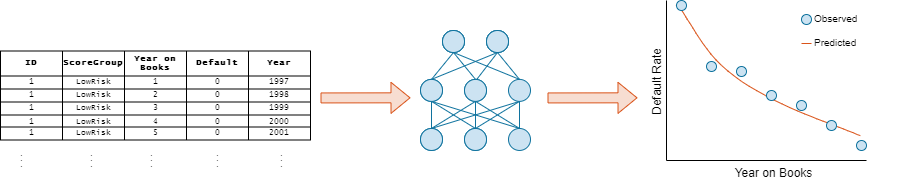In this example, you create and train three models for credit default prediction:

• Logistic regression network (also known as a single-layer perceptron)

• Multilayer perceptron (MLP)

• Residual network (ResNet)

You can express each of these models as a neural network of varying complexity and depth.

Load the retail credit panel data set. This data includes the following variables:

• `ID` — Loan identifier.

• `ScoreGroup` — Credit score at the beginning of the loan, discretized into three groups: `High Risk`, `Medium Risk`, and `Low Risk`.

• `YOB` — Years on books.

• `Default` — Default indicator. A value of `1` for `Default` means that the loan defaulted in the corresponding calendar year.

• `Year` — Calendar year.

```filename = fullfile(toolboxdir('risk'),'riskdata','RetailCreditPanelData.mat'); tbl = load(filename).data;```

### Encode Categorical Variables

To train a deep learning network, you must first encode the categorical `ScoreGroup` variable to one-hot encoded vectors.

View the order of the `ScoreGroup` categories.

`categories(tbl.ScoreGroup)'`
```ans = 1×3 cell {'High Risk'} {'Medium Risk'} {'Low Risk'} ```

Convert the categorical `ScoreGroup` variable to one-hot encoded vectors using the `onehotencode` function.

`riskGroup = onehotencode(tbl.ScoreGroup,2);`

Add the one-hot vectors to the table.

```tbl.HighRisk = riskGroup(:,1); tbl.MediumRisk = riskGroup(:,2); tbl.LowRisk = riskGroup(:,3);```

Remove the original `ScoreGroup` variable from the table using `removevars`.

`tbl = removevars(tbl,{'ScoreGroup'});`

Because you want to predict the `Default` variable response, move the `Default` variable to the end of the table.

`tbl = movevars(tbl,'Default','After','LowRisk');`

View the first few rows of the table. Notice that the `ScoreGroup` variable has been split into multiple columns, with the categorical values as the variable names.

`head(tbl)`
```ans=8×7 table ID YOB Year HighRisk MediumRisk LowRisk Default __ ___ ____ ________ __________ _______ _______ 1 1 1997 0 0 1 0 1 2 1998 0 0 1 0 1 3 1999 0 0 1 0 1 4 2000 0 0 1 0 1 5 2001 0 0 1 0 1 6 2002 0 0 1 0 1 7 2003 0 0 1 0 1 8 2004 0 0 1 0 ```

### Split Data

Partition the data set into training, validation, and test partitions using the unique loan ID numbers. Set aside 60% of the data for training, 20% for validation, and 20% for testing.

Find the unique loan IDs.

```idx = unique(tbl.ID); numObservations = length(idx);```

Determine the number of observations for each partition.

```numObservationsTrain = floor(0.6*numObservations); numObservationsValidation = floor(0.2*numObservations); numObservationsTest = numObservations - numObservationsTrain - numObservationsValidation;```

Create an array of random indices corresponding to the observations and partition it using the partition sizes.

```rng('default') idxShuffle = idx(randperm(numObservations)); idxTrain = idxShuffle(1:numObservationsTrain); idxValidation = idxShuffle(numObservationsTrain+1:numObservationsTrain+numObservationsValidation); idxTest = idxShuffle(numObservationsTrain+numObservationsValidation+1:end);```

Find the table entries corresponding to the data set partitions.

```idxTrainTbl = ismember(tbl.ID,idxTrain); idxValidationTbl = ismember(tbl.ID,idxValidation); idxTestTbl = ismember(tbl.ID,idxTest);```

Keep the variables of interest for the task (`YOB`, `HighRisk`, `MediumRisk`, `LowRisk`, and `Default`) and remove all other variables from the table.

```tbl = removevars(tbl,{'ID','Year'}); head(tbl)```
```ans=8×5 table YOB HighRisk MediumRisk LowRisk Default ___ ________ __________ _______ _______ 1 0 0 1 0 2 0 0 1 0 3 0 0 1 0 4 0 0 1 0 5 0 0 1 0 6 0 0 1 0 7 0 0 1 0 8 0 0 1 0 ```

Partition the table of data into training, validation, and testing partitions using the indices.

```tblTrain = tbl(idxTrainTbl,:); tblValidation = tbl(idxValidationTbl,:); tblTest = tbl(idxTestTbl,:);```

### Define Network Architectures

You can use different deep learning architectures for the task of predicting credit default probabilities. Smaller networks are quick to train, but deeper networks can learn more abstract features. Choosing a neural network architecture requires balancing computation time against accuracy. In this example, you define three network architectures, with varying levels of complexity.

#### Logistic Regression Network

The first network is a simple neural network containing four layers.

Start with a feature input layer, which passes tabular data (credit panel data) to the network. In this example, there are four input features: `YOB`, `HighRisk`, `MediumRisk`, and `LowRisk`. Configure the input layer to normalize the data using z-score normalization. Normalizing the data is important for tasks where the scale and range of the input variables is very different.

Next, use a fully connected layer with a single output followed by a sigmoid layer. For the final layer, use a custom binary cross-entropy loss layer. This layer is attached to this example as a supporting file.

```logisticLayers = [ featureInputLayer(4,'Normalization','zscore') fullyConnectedLayer(1) sigmoidLayer BinaryCrossEntropyLossLayer('output')];```

This network is called a single-layer perceptron. You can visualize the network using Deep Network Designer or the `analyzeNetwork` function.

`deepNetworkDesigner(logisticLayers)`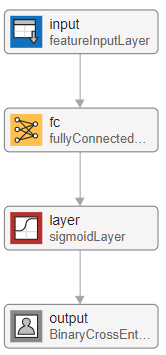You can easily show that the single-layer perceptron neural network is equivalent to logistic regression. Let ${x}_{i}$ be a 1-by-4 vector containing the features for observation $i$. With input ${x}_{i}$, the output of the fully connected layer is

$W{x}_{i}+b={W}_{1}{x}_{i,1}+{W}_{2}{x}_{i,2}+{W}_{3}{x}_{i,3}+{W}_{4}{x}_{i,4}+b$.

The output of the fully connected layer provides the input for the sigmoid layer. The sigmoid layer then outputs

$S\left(W{x}_{i}+b\right)=\frac{1}{1+\mathrm{exp}\left\{-\left({W}_{1}{x}_{i,1}+{W}_{2}{x}_{i,2}+{W}_{3}{x}_{i,3}+{W}_{4}{x}_{i,4}+b\right)\right\}}$.

The output of the sigmoid layer is equivalent to the logistic regression model. The final layer of the neural network is a binary cross-entropy loss layer. Minimizing the binary cross-entropy loss is equivalent to maximizing the likelihood in a logistic regression model.

#### Multilayer Perceptron

The next network has a similar architecture to the logistic regression model, but has an additional fully connected layer with an output size of 100, followed by a ReLU nonlinear activation function. This type of network is called a multilayer perceptron due to the addition of another hidden layer and a nonlinear activation function. Whereas the single-layer perceptron can learn only linear functions, the multilayer perceptron can learn complex, nonlinear relationships between the input and output data.

```mlpLayers = [ featureInputLayer(4,'Normalization','zscore') fullyConnectedLayer(100) reluLayer fullyConnectedLayer(1) sigmoidLayer BinaryCrossEntropyLossLayer('output')]; deepNetworkDesigner(mlpLayers)```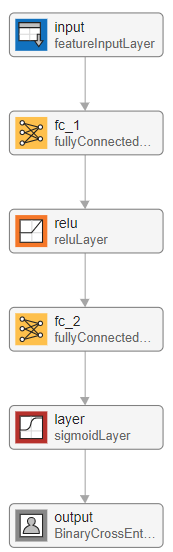#### Residual Network

For the final network, create a residual network (ResNet)  from multiple stacks of fully connected layers and ReLU activations. Originally developed for image classification, ResNets have proven successful across many domains. Because a ResNet has many more parameters than multilayer perceptrons or logistic networks, they take longer to train.

```residualLayers = [ featureInputLayer(4,'Normalization','zscore','Name','input') fullyConnectedLayer(16,'Name','fc1') batchNormalizationLayer('Name','bn1') reluLayer('Name','relu1') fullyConnectedLayer(32,'Name','resblock1-fc1') batchNormalizationLayer('Name','resblock1-bn1') reluLayer('Name','resblock1-relu1') fullyConnectedLayer(32,'Name','resblock1-fc2') additionLayer(2,'Name','resblock1-add') batchNormalizationLayer('Name','resblock1-bn2') reluLayer('Name','resblock1-relu2') fullyConnectedLayer(64,'Name','resblock2-fc1') batchNormalizationLayer('Name','resblock2-bn1') reluLayer('Name','resblock2-relu1') fullyConnectedLayer(64,'Name','resblock2-fc2') additionLayer(2,'Name','resblock2-add') batchNormalizationLayer('Name','resblock2-bn2') reluLayer('Name','resblock2-relu2') fullyConnectedLayer(1,'Name','fc2') sigmoidLayer('Name','sigmoid') BinaryCrossEntropyLossLayer('output')]; residualLayers = layerGraph(residualLayers); residualLayers = addLayers(residualLayers,fullyConnectedLayer(32,'Name','resblock1-fc-shortcut')); residualLayers = addLayers(residualLayers,fullyConnectedLayer(64,'Name','resblock2-fc-shortcut')); residualLayers = connectLayers(residualLayers,'relu1','resblock1-fc-shortcut'); residualLayers = connectLayers(residualLayers,'resblock1-fc-shortcut','resblock1-add/in2'); residualLayers = connectLayers(residualLayers,'resblock1-relu2','resblock2-fc-shortcut'); residualLayers = connectLayers(residualLayers,'resblock2-fc-shortcut','resblock2-add/in2'); deepNetworkDesigner(residualLayers)```

#### Network Depth

The depth of a network is an important concept in deep learning and is defined as the largest number of sequential convolutional or fully connected layers (represented by yellow blocks in the following diagram) on a path from the input layer to the output layer. The deeper a network is, the more complex features it can learn. In this example, the logistic network has a depth of 1, the multilayer perceptron has a depth of 2, and the residual network has a depth of 6.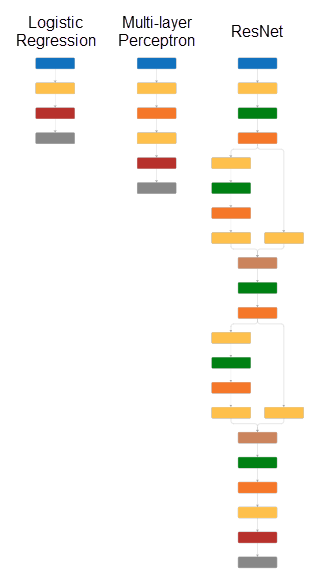### Specify Training Options

Specify the training options.

• Train using the Adam optimizer.

• Set the initial learning rate to 0.001.

• Set the mini-batch size to 512.

• Turn on the training progress plot and turn off the command window output.

• Shuffle the data at the beginning of each epoch.

• Monitor the network accuracy during training by specifying validation data and using it to validate the network every 1000 iterations.

```options = trainingOptions('adam', ... 'InitialLearnRate',0.001, ... 'MiniBatchSize',512, ... 'Plots','training-progress', ... 'Verbose',false, ... 'Shuffle','every-epoch', ... 'ValidationData',tblValidation, ... 'ValidationFrequency',1000);```

The loss landscape of the logistic regression network is convex, therefore, it does not need to train for as many epochs. For the logistic regression and multilayer perceptron models, train for 15 epochs. For the more complex residual network, train for 50 epochs.

```logisticOptions = options; logisticOptions.MaxEpochs = 15; mlpOptions = options; mlpOptions.MaxEpochs = 15; residualOptions = options; residualOptions.MaxEpochs = 50;```

The three networks have different architectures, so they require different sets of training options to achieve optimal performance. You can perform optimization programmatically or interactively using Experiment Manager. For an example showing how to perform a hyperparameter sweep of the training options, see Create a Deep Learning Experiment for Classification.

### Train Network

Train the networks using the architectures defined, the training data, and the training options. By default, `trainNetwork` uses a GPU if one is available; otherwise, it uses a CPU. Training on a GPU requires Parallel Computing Toolbox™ and a supported GPU device. For information on supported devices, see GPU Support by Release (Parallel Computing Toolbox). You can also specify the execution environment by using the `ExecutionEnvironment` name-value argument of `trainingOptions`.

To avoid waiting for training, load pretrained networks by setting the `doTrain` flag to `false`. To train the networks using `trainNetwork`, set the `doTrain` flag to `true`.

Training times using a NVIDIA® GeForce® RTX 2080 Ti are:

• Logistic network — Approximately 4 minutes

• Multilayer perceptron — Approximately 5 minutes

• Residual network — Approximately 35 minutes

```doTrain = false; if doTrain logisticNet = trainNetwork(tblTrain,'Default',logisticLayers,logisticOptions); mlpNet = trainNetwork(tblTrain,'Default',mlpLayers,mlpOptions); residualNet = trainNetwork(tblTrain,'Default',residualLayers,residualOptions); else load logisticTrainedNetwork load mlpTrainedNetwork load residualTrainedNetwork end```

### Test Network

Predict the default probability of the test data using the trained networks.

```tblTest.logisticPred = predict(logisticNet,tblTest(:,1:end-1)); tblTest.mlpPred = predict(mlpNet,tblTest(:,1:end-1)); tblTest.residualPred = predict(residualNet,tblTest(:,1:end-1));```

#### Default Rates by Year on Books

To assess the performance of the network, use the `groupsummary` function to group the true default rates and corresponding predictions by years on books (represented by the `YOB` variable) and calculate the mean value.

```summaryYOB = groupsummary(tblTest,'YOB','mean',{'Default','logisticPred','mlpPred','residualPred'}); head(summaryYOB)```
```ans=8×6 table YOB GroupCount mean_Default mean_logisticPred mean_mlpPred mean_residualPred ___ __________ ____________ _________________ ____________ _________________ 1 19364 0.017352 0.017471 0.018056 0.017663 2 18917 0.012158 0.014209 0.015486 0.014192 3 18526 0.011875 0.011538 0.013154 0.011409 4 18232 0.011683 0.0093902 0.011151 0.010311 5 17925 0.0082008 0.007626 0.0089826 0.0093438 6 17727 0.0066565 0.0062047 0.0062967 0.0073401 7 12294 0.0030909 0.0050643 0.0042998 0.0047071 8 6361 0.0017293 0.0041463 0.0029052 0.0025272 ```

Plot the true average default rate against the average predictions by years on books.

```networks = ["Logistic Regression Network","Multilayer Percerptron Network","Residual Network"]; figure tiledlayout('flow','TileSpacing','compact') for i = 1:3 nexttile scatter(summaryYOB.YOB,summaryYOB.mean_Default*100,'*'); hold on plot(summaryYOB.YOB,summaryYOB{:,i+3}*100); hold off title(networks(i)) xlabel('Years on Books') ylabel('Default Rate (%)') legend('Observed','Predicted') end```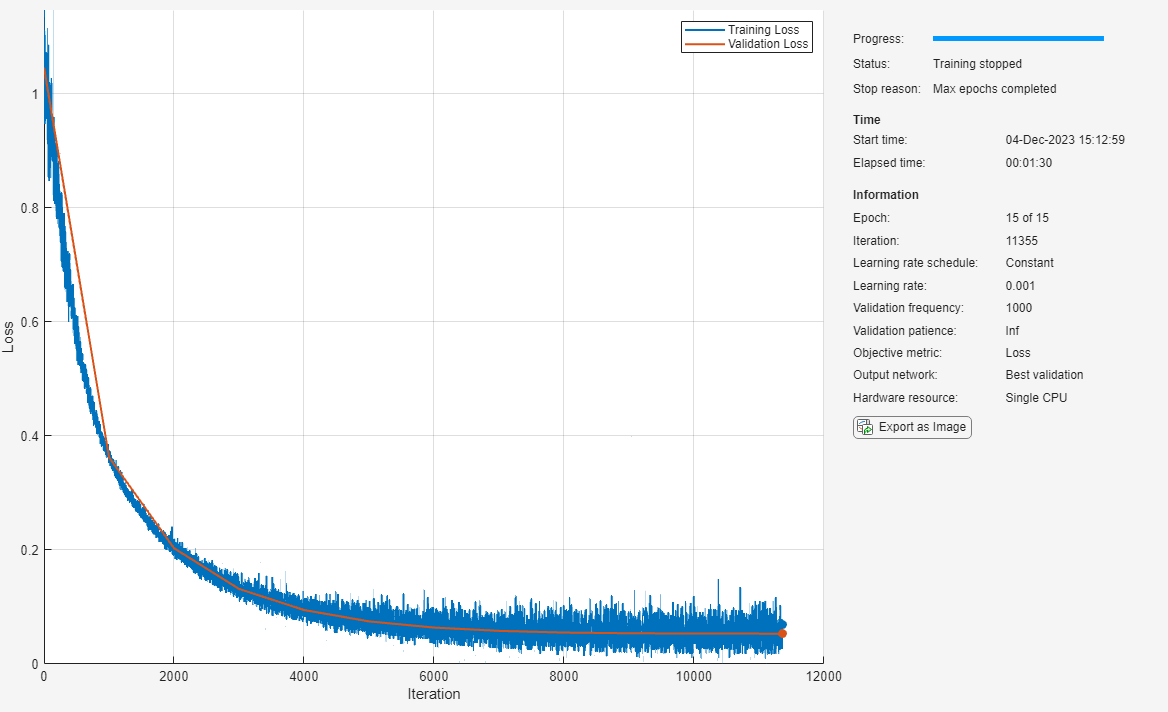All three networks show a clear downward trend, with default rates going down as the number of years on books increases. Years three and four are an exception to the downward trend. Overall, the three models predict the default rates well, and even the simpler logistic regression model predicts the general trend. The residual network captures a more complex, nonlinear relationship compared to the logistic model, which can fit only a linear relationship.

#### Default Rates by Score Groups

Use the credit score group as a grouping variable to compute the observed and predicted default rate for each score group.

Decode `ScoreGroup` back into the categorical score groups.

```ScoreGroup = onehotdecode(tblTest{:,2:4},{'HighRisk','MediumRisk','LowRisk'},2); tblTest.ScoreGroup = ScoreGroup; tblTest = removevars(tblTest,{'HighRisk','MediumRisk','LowRisk'}); riskGroups = categories(tblTest.ScoreGroup);```

Use the `groupsummary` function to group the true default rate and the predictions by `YOB` and `ScoreGroup`, and return the mean for each group.

```numYOB = height(summaryYOB); numRiskGroups = height(riskGroups); summaryYOBScore = groupsummary(tblTest,{'ScoreGroup','YOB'},'mean',{'Default','logisticPred','mlpPred','residualPred'}); head(summaryYOBScore)```
```ans=8×7 table ScoreGroup YOB GroupCount mean_Default mean_logisticPred mean_mlpPred mean_residualPred __________ ___ __________ ____________ _________________ ____________ _________________ HighRisk 1 6424 0.029577 0.028404 0.031563 0.02973 HighRisk 2 6180 0.020065 0.02325 0.026649 0.023655 HighRisk 3 5949 0.019163 0.019013 0.022484 0.018724 HighRisk 4 5806 0.020668 0.015535 0.018957 0.017207 HighRisk 5 5634 0.01349 0.012686 0.01577 0.015374 HighRisk 6 5531 0.013379 0.010354 0.010799 0.01215 HighRisk 7 3862 0.0051787 0.0084466 0.0071398 0.0083738 HighRisk 8 2027 0.0034534 0.0068881 0.0047145 0.0040559 ```

Plot the true average default rate against the predicted rate by years on books and risk group.

```figure t = tiledlayout('flow','TileSpacing','compact'); color = lines(3); YOB = summaryYOBScore.YOB; default = summaryYOBScore.mean_Default*100; group = summaryYOBScore.ScoreGroup; for i = 1:3 pred = summaryYOBScore{:,i+4}*100; meanScore = reshape(pred,numYOB,numRiskGroups); nexttile hs = gscatter(YOB,default,group,color,'*',6,false); hold on colororder(color) plot(meanScore) hold off title(networks(i)) xlabel('Years on Books') ylabel('Default Rate (%)') grid on end labels = ["True: " + riskGroups; "Pred: " + riskGroups]; lgd = legend(labels); lgd.Layout.Tile = 4;```The plot shows that all score groups behave similarly as time progresses, with a general downward trend. Across the high risk group, year four does not follow the downward trend. In the medium risk group, years three and four appear flat. Finally, in the low risk group, year three shows an increase. These irregular trends are difficult to discern with the simpler logistic regression model.

For an example showing how to use the locally-interpretable model-agnostic explanations (LIME) and Shapley values interpretability techniques to understand the predictions of a residual network for credit default prediction, see Interpret and Stress-Test Deep Learning Networks for Probability of Default (Risk Management Toolbox).

### References

 He, Kaiming, Xiangyu Zhang, Shaoqing Ren, and Jian Sun. "Deep residual learning for image recognition." In Proceedings of the IEEE conference on computer vision and pattern recognition, pp. 770-778. 2016.